Spherical Text Magnification

Spherical text magnification refers to a displacement technique that moves letter objects around the surface of a three-dimensional sphere:In sphericalMag.fla , the characters continually watch the position of the mouse and determine their own positions based on its proximity. If the mouse is outside a certain "sphere of effect," the character remains unchanged, sitting in its original position. Once the mouse enters the sphere of effect, the character is at first pushed away, then drawn in after the mouse reaches the halfway mark. This process of expansion and contraction is smoothed using a sinusoidal function.

Building the Spherical Text Magnifier

To create this system, you will prototype a reactive letter and instantiate a grid of them. As with the previous project, the motivation to build the movie in this way should be easy to understand: the prototype gives you the fastest performance possible in Flash MX while providing an easy-to-use modular framework.

1. First, open a new Flash movie and set the frame rate at a comfortable 30 fps. On frame 1 of the main timeline, add the following environmental constants. Your reactive letter objects will be referencing these variables later.

// register root as environment  Object.environment = this;  // spherical lens radius  this.frad = 100;  // spherical magnification amount  this.mag = 2;
2. Next, create a new movie clip and name it TextNode . Export it with the linkage identifier textNode . The TextNode is going to become your reactive letter object. Name the first layer definition and create a new layer named body .

3. On the body layer, create a new dynamic text field and assign it the variable txtWord . Set the style and size of the type as you desire . Now select the text field and convert it into a movie clip. Name the movie clip TextNodeBody ; it's not necessary to export it (the reason you are not exporting this movie clip is because you will not be attaching it dynamically and it contains no prototyped functions). Now highlight the instance of TextNodeBody that already exists on the body layer of TextNode . Give it an instance name of body . You can do this by typing the word body into the <instance name> field of the Property Inspector. The reason you are subclassing the text field is so that you can scale it while keeping the TextNode movie clip unaffected.

4. On the layer definition of the TextNode movie clip, prototype the movie clip in the usual fashion by typing the following on frame 1 (you can also see this code in sphericalMag.fla ):

#initclip  // build the TextNode object  function TextNode() {   this.setup();   }  // inherit movie clip object properties  TextNode.prototype = new MovieClip();  // instance functions...  TextNode.prototype.setup = function() {  // set random character  this.body.ch = String.fromCharCode(random(26)+97);  // set size of body  this.body._xscale=this.bodysize;   this.body._yscale=this.bodysize;  // watch mouse for lifetime  this.onEnterFrame = this.render;   };   TextNode.prototype.render = function() {  // watch the mouse - move and scale accordingly  var dx = Object.environment  .  _xmouse-this.x;   var dy = Object.environment._ymouse-this.y;  // calculate distance to mouse  var d = Math.sqrt(dx*dx+dy*dy);  // if distance is within 'sphere of effect'  if (d>=Object.environment.frad) {  // beyond edge of sphere, text remains where it should  this._x = this.x;   this._y = this.y;   } else {  // within the sphere, conjure from the depths of number  var lensDisp = Math.sin(Math.PI*Math.abs(d/this._parent.frad));  // position the text  this._x = this.x-dx*lensDisp;   this._y = this.y-dy*lensDisp;   var lensMag = Object.environment.mag*(1-Math.sin(Math.PI *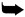Math.abs(d/Object.environment.frad)/2));   }   this.body._xscale = this.bodySize*(lensMag+1);   this.body._yscale = this.bodySize*(lensMag+1);   };  // register class  Object.registerClass("textNode", TextNode);   #endinitclip
5. The construction and initialization of this movie clip is probably familiar by now. In the prototyping style, you begin the definition of the movie clip with a constructing function that calls the setup function. Let's take a look at the essential parts of the setup function:

// set random character     this.body.ch = String.fromCharCode(random(26)+97);

This code sets the text field in the body movie clip to some random character, from a to z.

// set size of body     this.body._xscale=this.bodysize;     this.body._yscale=this.bodysize;

Here you are setting the visual size of the movie clip by resizing the small body contained within it. This will be important later, when you add elements to the TextNode that you do not want to be scaled.

// watch mouse for lifetime     this.onEnterFrame = this.render;

This final line sets the behavior of the TextNode for the rest of its existence, as defined in the render function.

6. Next up, the render function keeps an eye on the proximity of the mouse and does all the serious mathematical work of the three-dimensional transformations. When the mouse enters a given range set by the environmental constant frad , the TextNode displaces and scales itself so as to appear on the edge of a moving sphere.

The following diagram shows the behavior of render as a function of the mouse's position. The outer circle represents the active radius set by frad . The inner circle represents the extent to which the letter will travel as the mouse nears. The middle circle represents the halfway mark of frad . Although it is true the letter is displaced from its actual coordinates, notice that in both the first and last positions the letter is precisely where it should be. This can happen because the letter recedes from the mouse until the halfway mark. Once more than halfway there, the letter approaches the mouse. The letter increases in size spherically proportional to the approach. At full size the letter is bodysize (the original scale) multiplied by mag .

Let's look at the individual elements of the render function to learn how it works:// watch the mouse - move and scale accordingly     var dx = Object.environment._xmouse-this.x;     var dy = Object.environment._ymouse-this.y;     // calculate distance to mouse     var d = Math.sqrt(dx*dx+dy*dy);

This is a simple calculation of distance (in pixels) between the mouse and the object itself.

// if distance is within 'sphere of effect'     if (d>=Object.environment.frad) {       // beyond edge of sphere, text remains where it should       this._x = this.x;       this._y = this.y;     } else {

Here you compare the distance to the mouse. If it is greater than the environmental constant frad , the position and scale calculations are easy because the letter is outside the sphere of effect. Otherwise, the letter's position and scale need to be modified.

Calculation of the position and scale of the letter involves two sinusoidal curves, two environmental constants, the fractional position of the mouse within the sphere of effect, and some faith.

// within the sphere, conjure from the depths of number       var lensDisp = Math.sin(Math.PI*Math.abs(d/this._parent.frad  ));

First, you calculate the displacement intensity as a factor of how close the mouse is to the letter's center. This intensity curves along a sinusoidal arc so the letter appears to move across a sphere, rather than a pyramid. You store this value as lensDisp .

// position the text      this._x = this.x - dx * lensDisp;      this._y = this.y - dy * lensDisp;

Next, you use the displacement intensity as a factor in the positioning of the letter. Starting from where the letter should be, the new position is the difference computed by multiplying the mouse distance by the displacement intensity. As you will see later in this section, these two lines offer great potential for interesting modification.

var lensMag = Object.environment.mag*(1-Math.sin(Math.PI *Math.abs(d/Object.environment.frad)/2));     }

As a final step for letters within the sphere of effect, you compute a value that will be used in the sizing of the letter. This is essentially the same equation used in lensDisp , except you want letters to continue to grow larger the entire time the mouse approaches. This requires an extra divisor of 2.

this.body._xscale = this.bodySize*(lensMag+1);     this.body._yscale = this.bodySize*(lensMag+1);    };

Here you set the scale of the body movie clip to the size it should be, multiplied by the value of lensMag plus 1.

Earlier, you created two global constants called frad and mag . These constants control the size and intensity of the spherical magnification effect. The first, frad , sets the radius of the magnification in pixels. Thus, a frad value of 100 would produce a spherical magnification 200 pixels wide. The other constant, mag , sets the magnification level as a multiplying factor. Thus, a mag value of 4.2 would create a spherical magnification of 4.2x, and characters directly under the mouse will appear 420% as large as normal (it's worth noting that negative mag values produce weird results).

7. Now you'll write a nested loop that creates a grid of TextNode objects. A nested for loop running across the rows and columns of the grid should do the trick. Place the following code in the first frame of the main timeline, just underneath the environmental constants that you defined in step 1 of this tutorial:

// creation and positioning of 100 characters  for (gy=0; gy<10; gy++) {   for (gx=0; gx<10; gx++) {  // make a letter centered at this grid spot       // name the new text node  nombre = "tn"+String(depth++);  // initialize the text node with the parameters passed  posy = 200+gy*20;   posx = 200+gx*20;   init = {_x:posx, _y:posy, x:posx, y:posy, bodysize:25};  // attach the text node  this.attachMovie("textNode", nombre, depth, init);   }   }   stop();

Notice how each letter is created and initialized immediately after being attached. A stop() action at the end of the loop indicates that you are done working. The movie is now ready to test (CTRL/CMD+ENTER.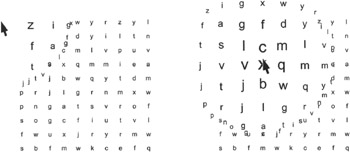The effect is a seemingly static field of characters, arranged in a grid. Upon closer inspection with your mouse cursor, the letters jump out of their positions, accelerating around the edge of an invisible sphere until they arrive , magnified, under the position of the mouse. When seen in motion, the behavior is very intuitive, and the process of scanning and reading the information can be quickly mastered.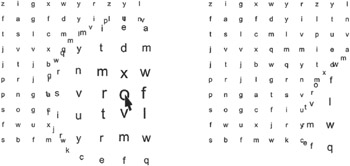Again, we'll look at a few different ways of extending this project in the subsections that follow.

Line to Origin

Adding a simple function that draws a line to the origin of the movie clip further demonstrates the three-dimensional processes used to move it around (extending the line in the other direction is interesting to look at, but not so useful).

1. Somewhere inside the render function on the definition layer of the TextNode movie clip, add the following function call (see sphericalMag_line.fla for further details):

this.drawLineToOrigin();
2. Prototype this new function by adding the following to the TextNode movie clip definition:

TextNode.prototype.drawLineToOrigin = function() {   this.clear();   this.lineStyle(0, 0x000000, 15);   this.lineTo(this.x-this._x, this.y-this._y);   };
3. And that's it! Go ahead and test your new, improved version of this movie. You really get an enhanced sense of depth with this iteration of the effect.Weird Lens

As mentioned earlier, there are two lines in the calculation of the TextNode displacement that you can have a lot of fun with. Keep in mind the calculations used to move the letter around the edge of a sphere, and take a look at sphericalMag_weird.fla . In this file, we have doubled the effect of the lens displacement by including an extra constant of 2:

...           //  .  within the sphere, conjure from the depths of number           var lensDisp = Math.sin(Math.PI*Math.abs(d/this._parent.frad));           // position the text           this._x = this.x - dx * lensDisp  * 2;  this._y = this.y - dy * lensDisp * 2;           ...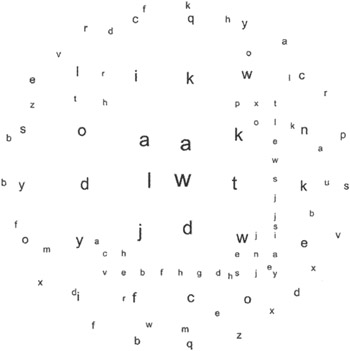Additionally, including multiples to the calculating of the displacement intensity creates wavy effects. These changes are available in sphericalMag_weird2.fla . To create this file on your own, begin with the original spherical magnification framework, and then change the calculation of lensDisp within the TextNode movie clip to the following:

// within the sphere, conjure from the depths of number           var lensDisp = Math.sin(Math.PI*Math.abs(d/this._parent.frad)*4) ;

In essence, this creates a field of text letters where each letter approaches and recedes from the mouse four times after entering the sphere of influence. Moving the mouse about the stage causes quite a stir of computational frenzy. The resulting animation looks like a swarm of letter insects attacking the cursor: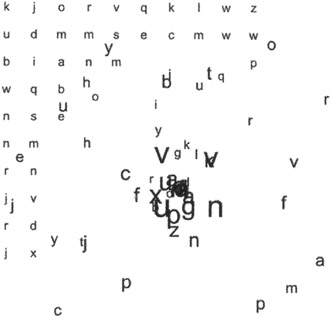Flash 3D Cheats Most Wanted
ISBN: 1590592212
EAN: N/A
Year: 2002
Pages: 97